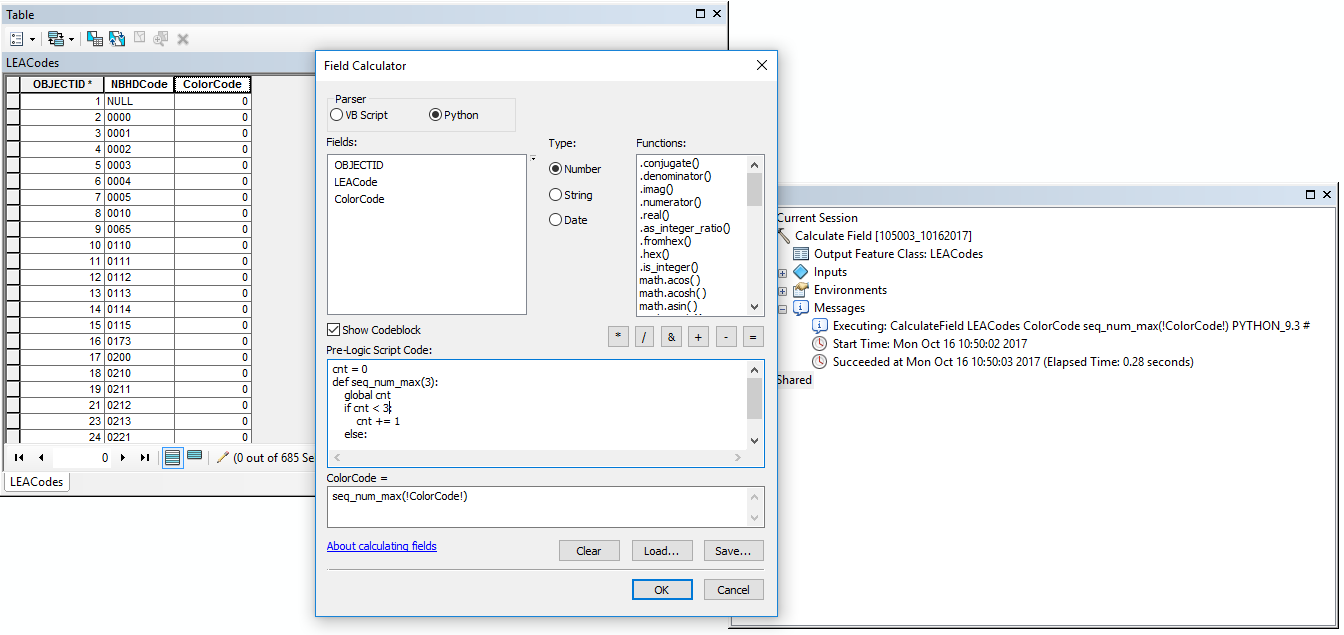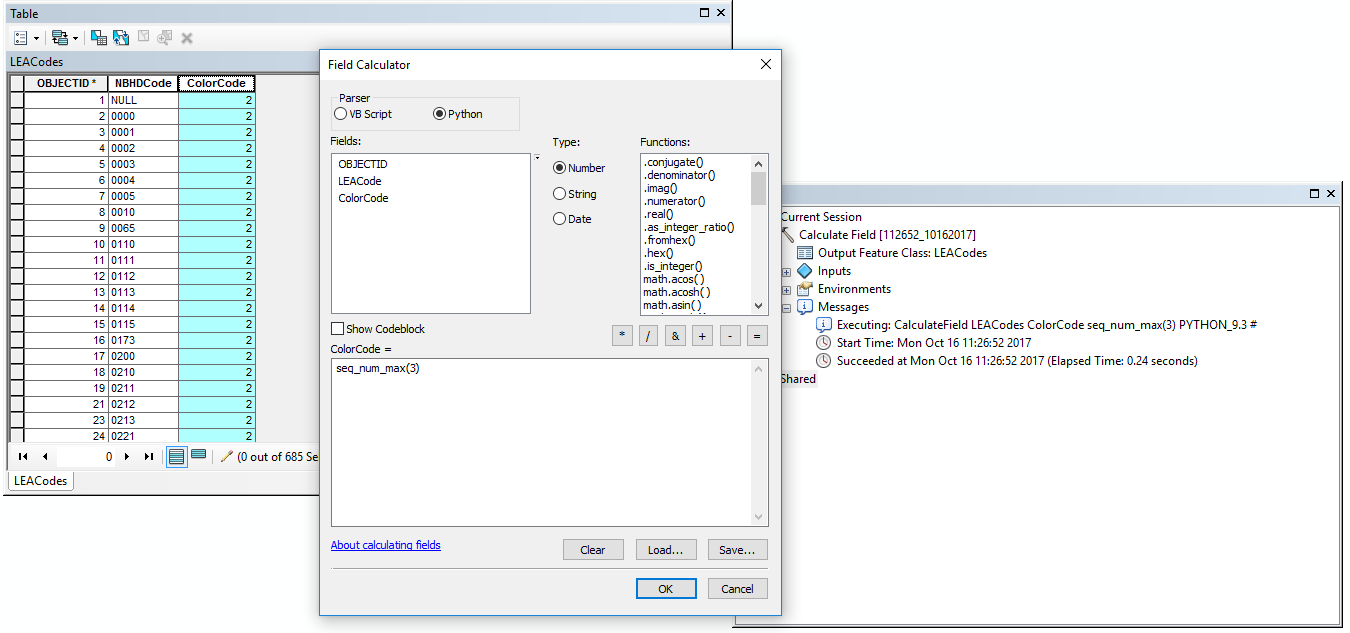# Calculate repeating values in field

1691
12
10-13-2017 03:48 PMNew Contributor III

Anyone have any ideas how to calculate a field so the values (going down the table) are

1

2

3

1

2

3

1

2

3

etc. to the end of the table?

These values will end up being color codes for rendering.  The actual values will be from 0-9, but need some way to get the repeating values in.  Another option might be to calculate random values instead of repeating values.  Any thoughts?

Tags (2)
1 Solution

Accepted SolutionsbyMVP Honored Contributor

This passes the current field value to the function (not what you want - would pass all 0s first time, then all 1s, etc.):

``seq_num_max(!ColorCode!)‍‍``

Try (you always want 3):

``seq_num_max(3)‍‍‍``

And code block (untested):

``````cnt = 0
def seq_num_max(val):
global cnt
if cnt < val:
cnt += 1
else:
cnt = 0
return cnt``````

Or (untested):

``````cnt = -1
def seq_num_max(val):
global cnt
cnt += 1
return (cnt % val) + 1‍‍‍‍‍``````
12 RepliesbyMVP Legendary Contributor
``````cnt = 0
def seq_num_max(val):
global cnt
if cnt < val:
cnt += 1
else:
cnt = 0
return cnt

# in expression box ... seq_num_max(3) ... will number from 0 to 3 before repeating‍‍‍‍‍‍‍‍‍‍``````

will get you started ... for use in the field calculator obviouslyOccasional Contributor III

I think this would do it:

In field calculator Codeblock (Python):

``````def Calculate(repeatingValue):
interval = 1
if cntr < 3:
cntr += interval
else:
cntr = 1
return cntr‍‍‍‍‍‍‍‍‍‍‍‍‍‍‍‍``````

Then in your expression it would be Calculate(!YourFieldName!)

MicahbyMVP Honored Contributor
``````for i in range(10):
print (i % 3) + 1

1
2
3
1
2
3
1
2
3
1‍‍‍‍‍‍‍‍‍‍‍‍‍``````New Contributor III

Thanks for your replies.  Dan, Micah, each of your code pieces seems to run well, but the values are not populating in the highlighted field.  All rows remain Null--see below.  Ideas?Occasional Contributor III

Hey Jeff,

You'll need to apply that function in the main calc expression box:

seq_num_max(!ColorCode!)

That should do it.New Contributor III

I am now getting all zero's in the ColorCode field.  Am I setting up the Field Calculator dialog correctly?  Or maybe something else?New Contributor III

Each time I run this code:

``````cnt = 0
def seq_num_max(val):
global cnt
if cnt < val:
cnt += 1
else:
cnt = 0
return cnt``````

The result calculates ALL values to 0, then to 1, then to 2, then 3, then back to 0 again, and so on.  Still not sure how to get the repeating values?byMVP Honored Contributor

This passes the current field value to the function (not what you want - would pass all 0s first time, then all 1s, etc.):

``seq_num_max(!ColorCode!)‍‍``

Try (you always want 3):

``seq_num_max(3)‍‍‍``

And code block (untested):

``````cnt = 0
def seq_num_max(val):
global cnt
if cnt < val:
cnt += 1
else:
cnt = 0
return cnt``````

Or (untested):

``````cnt = -1
def seq_num_max(val):
global cnt
cnt += 1
return (cnt % val) + 1‍‍‍‍‍``````New Contributor III

And Darren hits the grand slam . . . that worked!  Thank you!

You gave two suggestions--the second one did the trick.

Thanks to all who shared your ideas.